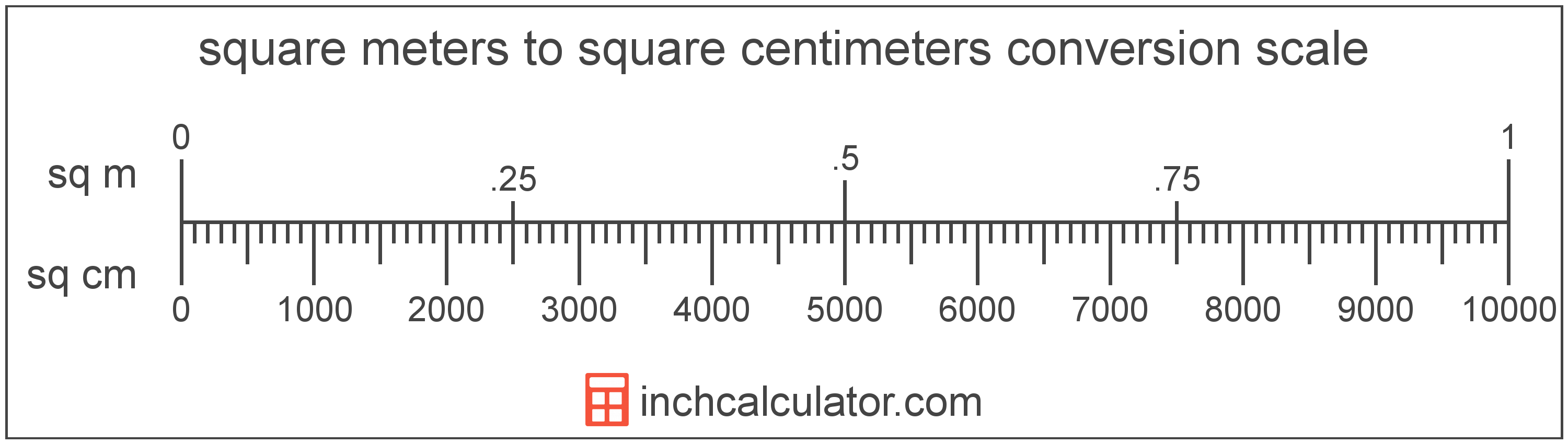# Square Centimeters to Square Meters Conversion

Enter the area in square centimeters below to get the value converted to square meters.

Results in Square Meters:1 sq cm = 0.0001 sq m

## How to Convert Square Centimeters to Square MetersTo convert a square centimeter measurement to a square meter measurement, divide the area by the conversion ratio.

Since one square meter is equal to 10,000 square centimeters, you can use this simple formula to convert:

square meters = square centimeters ÷ 10,000

The area in square meters is equal to the square centimeters divided by 10,000.

For example, here's how to convert 500 square centimeters to square meters using the formula above.
500 sq cm = (500 ÷ 10,000) = 0.05 sq m

## Square Centimeters

One square centimeter is equal to the area of a square with 1 centimeter long sides.

The square centimeter, or square centimetre, is a multiple of the square meter, which is the SI derived unit for area. In the metric system, "centi" is the prefix for 10-2. A square centimeter is sometimes also referred to as a square cm. Square centimeters can be abbreviated as sq cm, and are also sometimes abbreviated as cm². For example, 1 square centimeter can be written as 1 sq cm or 1 cm².

## Square Meters

One square meter is equal to the area of a square with sides that are 1 meter in length.

The square meter, or square metre, is the SI derived unit for area in the metric system. A square meter is sometimes also referred to as a square m. Square meters can be abbreviated as sq m, and are also sometimes abbreviated as . For example, 1 square meter can be written as 1 sq m or 1 m².

Try our square meters calculator to calculate the area of a space.

## Square Centimeter to Square Meter Conversion Table

Square centimeter measurements converted to square meters
Square Centimeters Square Meters
1 sq cm 0.0001 sq m
2 sq cm 0.0002 sq m
3 sq cm 0.0003 sq m
4 sq cm 0.0004 sq m
5 sq cm 0.0005 sq m
6 sq cm 0.0006 sq m
7 sq cm 0.0007 sq m
8 sq cm 0.0008 sq m
9 sq cm 0.0009 sq m
10 sq cm 0.001 sq m
20 sq cm 0.002 sq m
30 sq cm 0.003 sq m
40 sq cm 0.004 sq m
50 sq cm 0.005 sq m
60 sq cm 0.006 sq m
70 sq cm 0.007 sq m
80 sq cm 0.008 sq m
90 sq cm 0.009 sq m
100 sq cm 0.01 sq m
200 sq cm 0.02 sq m
300 sq cm 0.03 sq m
400 sq cm 0.04 sq m
500 sq cm 0.05 sq m
600 sq cm 0.06 sq m
700 sq cm 0.07 sq m
800 sq cm 0.08 sq m
900 sq cm 0.09 sq m
1,000 sq cm 0.1 sq m
2,000 sq cm 0.2 sq m
3,000 sq cm 0.3 sq m
4,000 sq cm 0.4 sq m
5,000 sq cm 0.5 sq m
6,000 sq cm 0.6 sq m
7,000 sq cm 0.7 sq m
8,000 sq cm 0.8 sq m
9,000 sq cm 0.9 sq m
10,000 sq cm 1 sq m

## References

1. Collins Dictionary, Definition of 'square meter', https://www.collinsdictionary.com/us/dictionary/english/square-meter